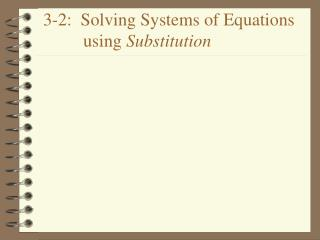DownloadDownload Presentation3-2: Solving Systems of Equations using Substitution

# 3-2: Solving Systems of Equations using Substitution

Download Presentation## 3-2: Solving Systems of Equations using Substitution

- - - - - - - - - - - - - - - - - - - - - - - - - - - E N D - - - - - - - - - - - - - - - - - - - - - - - - - - -
##### Presentation Transcript

1. 3-2: Solving Systems of Equations using Substitution

2. Solving Systems of Equations using Substitution Steps: 1. Solve one equation for one variable (y= ; x= ; a=) 2. Substitute the expression from step one into the other equation. 3. Simplify and solve the equation. 4. Substitute back into either original equation to find the value of the other variable. 5. Check the solution in both equations of the system.

3. Example #1: y = 4x 3x + y = -21 Step 1:Solve one equation for one variable. y = 4x(This equation is already solved for y.) Step 2: Substitute the expression from step one into the other equation. 3x + y = -21 3x + 4x = -21 Step 3: Simplify and solve the equation. 7x = -21 x = -3

4. y = 4x 3x + y = -21 Step 4: Substitute back into either original equation to find the value of the other variable. 3x + y = -21 3(-3) + y = -21 -9 + y = -21 y = -12 Solution to the system is (-3, -12).

5. y = 4x 3x + y = -21 Step 5: Check the solution in both equations. Solution to the system is (-3,-12). 3x + y = -21 3(-3) + (-12) = -21 -9 + (-12) = -21 -21= -21 y = 4x -12 = 4(-3) -12 = -12

6. Example #2: x + y = 10 5x – y = 2 Step 1: Solve one equation for one variable. x + y = 10 y = -x +10 Step 2: Substitute the expression from step one into the other equation. 5x - y = 2 5x -(-x +10) = 2

7. x + y = 10 5x – y = 2 Step 3: Simplify and solve the equation. 5x -(-x + 10) = 2 5x + x -10 = 2 6x -10 = 2 6x = 12 x = 2

8. x + y = 10 5x – y = 2 Step 4: Substitute back into either original equation to find the value of the other variable. x + y = 10 2 + y = 10 y = 8 Solution to the system is (2,8).

9. x + y = 10 5x – y = 2 Step 5: Check the solution in both equations. Solution to the system is (2, 8). 5x – y = 2 5(2) - (8) = 2 10 – 8 = 2 2 = 2 x + y =10 2 + 8 =10 10 =10